Burcin Yazgi Walsh, Chris Brunsdon, Martin Charlton

NCG, Maynooth University

Ireland clusters are obtained using the 2016 Census Data at Small Areas Level by following the instructions prepared by Chris Brunsdon, Jan Rigby and Martin Charlton for 2011 Ireland Census Data.

For the previous study done for 2011 Ireland Census Data you can check this link (https://rpubs.com/chrisbrunsdon/14998).

The original census data can be found through the link below (http://www.cso.ie/en/census/census2016reports/census2016smallareapopulationstatistics/).

CreateVariables <- function(SA2016, na.rm=TRUE) {
attach(SA2016)
Age0_4    <- 100 * ( T1_1AGE0T + T1_1AGE1T + T1_1AGE2T + T1_1AGE3T + T1_1AGE4T ) / T1_1AGETT
Age5_14   <- 100 * ( T1_1AGE5T + T1_1AGE6T + T1_1AGE7T + T1_1AGE8T + T1_1AGE9T +
T1_1AGE10T + T1_1AGE11T + T1_1AGE12T + T1_1AGE13T + T1_1AGE14T) / T1_1AGETT
Age25_44  <- 100 * ( T1_1AGE25_29T + T1_1AGE30_34T + T1_1AGE35_39T + T1_1AGE40_44T ) / T1_1AGETT
Age45_64  <- 100 * ( T1_1AGE45_49T + T1_1AGE50_54T + T1_1AGE55_59T + T1_1AGE60_64T ) / T1_1AGETT
Age65over <- 100 * ( T1_1AGE65_69T + T1_1AGE70_74T + T1_1AGE75_79T + T1_1AGE80_84T + T1_1AGEGE_85T ) / T1_1AGETT

EU_National           <- 100 * (T2_1UKN + T2_1PLN + T2_1LTN + T2_1EUN) / T2_1TN
ROW_National          <- 100 * (T2_1RWN) / T2_1TN
Born_outside_Ireland  <- 100 * (T2_1TBP - T2_1IEBP) / T2_1TBP

Separated            <- 100 * (T1_2SEPT + T1_2DIVT) / T1_2T
SinglePerson         <- 100 * (T5_2_1PP - T4_5RP  ) / T5_2_TP
Pensioner            <- 100 * T4_5RP / T4_5TP
LoneParent           <- 100 * (T4_3FOPFCT + T4_3FOPMCT) / T4_5TF
DINK                 <- 100 * T4_5PFF / T4_5TF
NonDependentKids     <- 100 * T4_4AGE_GE20F / T4_4TF

RentPublic         <- 100 * T6_3_RLAH / T6_3_TH
RentPrivate        <- 100 * T6_3_RPLH / T6_3_TH

Flats              <- 100 * T6_1_FA_H / T6_1_TH
NoCenHeat          <- 100 * T6_5_NCH / T6_5_T
RoomsHH            <- (T6_4_1RH + 2*T6_4_2RH + 3*T6_4_3RH + 4*T6_4_4RH + 5*T6_4_5RH + 6*T6_4_6RH + 7*T6_4_7RH +     8*T6_4_GE8RH) / T6_4_TH
PeopleRoom         <- T1_1AGETT / (T6_4_1RH + 2*T6_4_2RH + 3*T6_4_3RH + 4*T6_4_4RH + 5*T6_4_5RH + 6*T6_4_6RH + 7*T6_4_7RH + 8*T6_4_GE8RH)
SepticTank         <- 100 * T6_7_IST / T6_7_T

HEQual             <- 100 * ((T10_4_ODNDT + T10_4_HDPQT + T10_4_PDT + T10_4_DT) / T10_4_TT)
Employed           <- 100 * T8_1_WT / T8_1_TT
TwoCars            <- 100 * (T15_1_2C + T15_1_3C + T15_1_GE4C) / (T15_1_NC + T15_1_1C + T15_1_2C + T15_1_3C + T15_1_GE4C + T15_1_NSC)
JTWPublic          <- 100 * (T11_1_BUW + T11_1_TDLW) / T11_1_TW
HomeWork           <- 100 * T9_2_PH / T9_2_PT
LLTI               <- 100 * (T12_3_BT + T12_3_VBT) / T12_3_TT
UnpaidCare         <- 100 * T12_2_T / T1_1AGETT

Students           <- 100 * T8_1_ST / T8_1_TT
Unemployed         <- 100 * T8_1_ULGUPJT / T8_1_TT

EconInactFam       <- 100 * T8_1_LAHFT / T8_1_TT
Agric              <- 100 * T14_1_AFFT / T14_1_TT
Construction       <- 100 * T14_1_BCT / T14_1_TT
Manufacturing      <- 100 * T14_1_MIT / T14_1_TT
Commerce           <- 100 * T14_1_CTT / T14_1_TT
Transport          <- 100 * T14_1_TCT / T14_1_TT
Public             <- 100 * T14_1_PAT / T14_1_TT
Professional       <- 100 * T14_1_PST / T14_1_TT

#MISC
Broadband          <- 100 * T15_3_B / (T15_3_B + T15_3_OTH) # Internet connected HH with Broadband
Internet           <- 100 * (T15_3_B + T15_3_OTH) / T15_3_T # Households with Internet

Place <- data.frame(substring(GEOGID, 8), stringsAsFactors=FALSE)
detach(SA2016)

### Bringing it all together
colnames(Place) <- 'GEOGID'
Demographic <- data.frame(Age0_4, Age5_14, Age25_44, Age45_64, Age65over, EU_National, ROW_National, Born_outside_Ireland)
HouseholdComposition <- data.frame(Separated, SinglePerson, Pensioner, LoneParent, DINK, NonDependentKids)
Housing <- data.frame(RentPublic, RentPrivate, Flats, NoCenHeat, RoomsHH, PeopleRoom, SepticTank)
SocioEconomic <- data.frame(HEQual, Employed, TwoCars, JTWPublic, HomeWork, LLTI, UnpaidCare)
Employment <- data.frame(Students, Unemployed, EconInactFam, Agric, Construction, Manufacturing, Commerce, Transport, Public, Professional)

DerivedData <- data.frame(Place, Demographic, HouseholdComposition, Housing, SocioEconomic, Employment, Misc)
if (na.rm) DerivedData[which(is.na(DerivedData),arr.ind=T)] <- 0

DerivedData
}

After organising the data, we will be reading the data and computing the derived varaibles as explained above. At the end of these steps, we will have a working data set.

SAData <- read.csv(file="SAPS2016_SA2017.csv", header = TRUE, sep=",", stringsAsFactors=FALSE)
SAVars <- CreateVariables(SAData)

Cluster Analysis

We will be using the k-means cluster analysis ( 1 ) and as a first step we will be running the principal component analysis. After learning the proportion of the each component’s variance explanation, we will be investigating the cumulative effect.

PCA <- princomp(SAVars[, -1], cor = T, scores = T)
PCA$sdev^2/sum(PCA$sdev^2)
##       Comp.1       Comp.2       Comp.3       Comp.4       Comp.5
## 0.2484693804 0.1421954394 0.0891606551 0.0758493378 0.0400537948
##       Comp.6       Comp.7       Comp.8       Comp.9      Comp.10
## 0.0332321155 0.0308810401 0.0267886445 0.0245470766 0.0236552256
##      Comp.11      Comp.12      Comp.13      Comp.14      Comp.15
## 0.0215870004 0.0199187591 0.0191543176 0.0181670433 0.0170903608
##      Comp.16      Comp.17      Comp.18      Comp.19      Comp.20
## 0.0164860334 0.0148776432 0.0137297064 0.0127655558 0.0114793411
##      Comp.21      Comp.22      Comp.23      Comp.24      Comp.25
## 0.0105019385 0.0104688446 0.0091711753 0.0088711172 0.0079172581
##      Comp.26      Comp.27      Comp.28      Comp.29      Comp.30
## 0.0070287236 0.0064817850 0.0063391058 0.0054675320 0.0048938867
##      Comp.31      Comp.32      Comp.33      Comp.34      Comp.35
## 0.0042540572 0.0039313291 0.0028685743 0.0025762038 0.0024743384
##      Comp.36      Comp.37      Comp.38      Comp.39      Comp.40
## 0.0023213324 0.0016691996 0.0014561298 0.0009091458 0.0003098518
cumsum(PCA$sdev^2/sum(PCA$sdev^2))
##    Comp.1    Comp.2    Comp.3    Comp.4    Comp.5    Comp.6    Comp.7
## 0.2484694 0.3906648 0.4798255 0.5556748 0.5957286 0.6289607 0.6598418
##    Comp.8    Comp.9   Comp.10   Comp.11   Comp.12   Comp.13   Comp.14
## 0.6866304 0.7111775 0.7348327 0.7564197 0.7763385 0.7954928 0.8136598
##   Comp.15   Comp.16   Comp.17   Comp.18   Comp.19   Comp.20   Comp.21
## 0.8307502 0.8472362 0.8621139 0.8758436 0.8886091 0.9000885 0.9105904
##   Comp.22   Comp.23   Comp.24   Comp.25   Comp.26   Comp.27   Comp.28
## 0.9210593 0.9302304 0.9391015 0.9470188 0.9540475 0.9605293 0.9668684
##   Comp.29   Comp.30   Comp.31   Comp.32   Comp.33   Comp.34   Comp.35
## 0.9723360 0.9772298 0.9814839 0.9854152 0.9882838 0.9908600 0.9933343
##   Comp.36   Comp.37   Comp.38   Comp.39   Comp.40
## 0.9956557 0.9973249 0.9987810 0.9996901 1.0000000

As a result of the cumulative amount of the variance explained by the components, it is noticable that the first 14 components can explain the 81.3% of the variance in total. We will be using this number for k-means cluster analysis. Note that the number of principal component considered should be updated based on your own analysis.

smallest.clus <- wss <- rep(0, 100)
for (i in 1:100) {
clus <- kmeans(PCA$scores[, 1:14], i, iter.max = 100, nstart = 20) wss[i] <- clus$tot.withinss
smallest.clus[i] <- min(clus$size) } plot(1:100, wss[1:100], type = "h", main = "Cluster Scree Plot", xlab = "Number of Clusters", ylab = "Within Cluster Sum of Squares")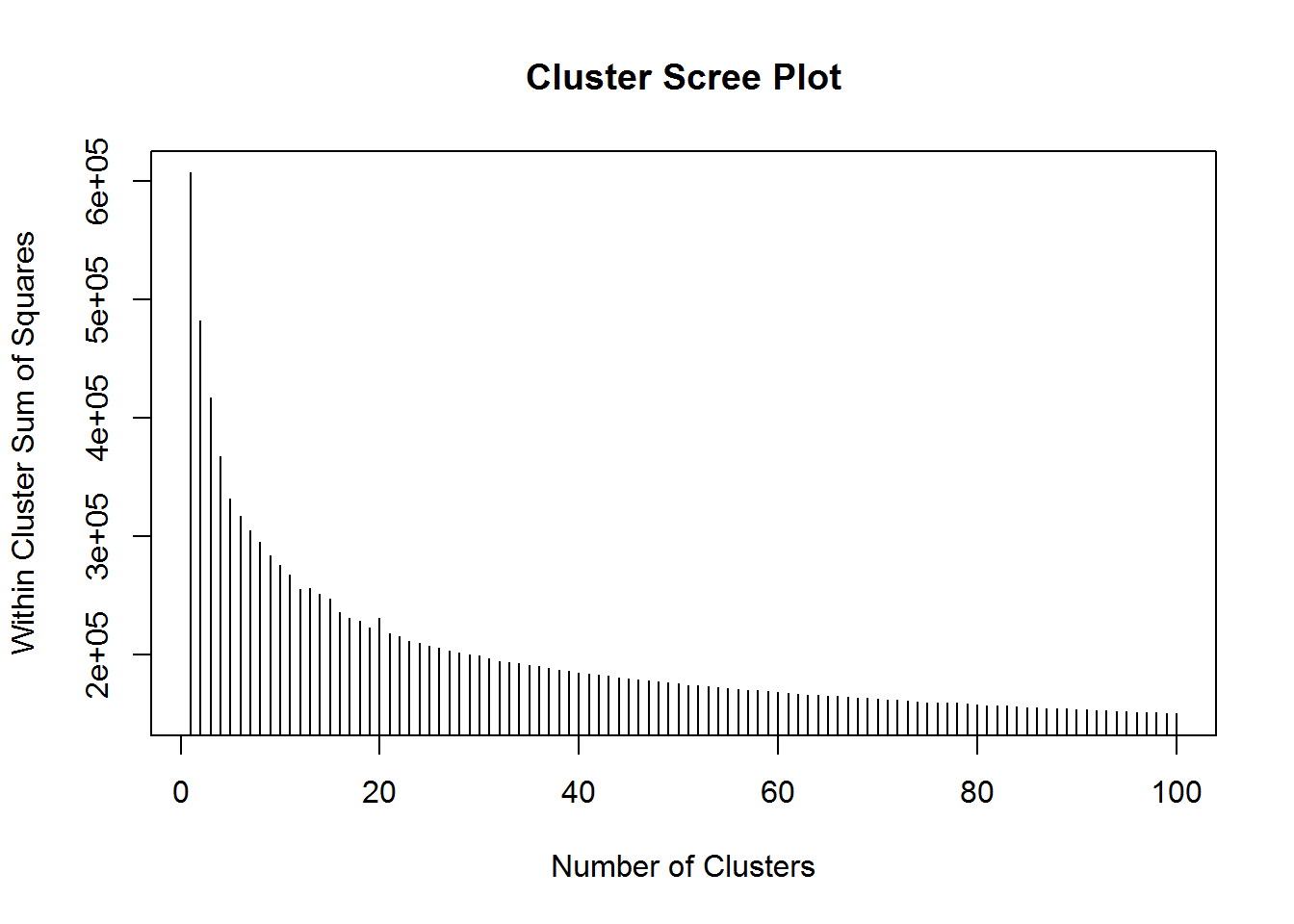“A further issue is the size of the clusters. One problem frequently occuring with ( k )-means cluster analysis is that very unusual cases tend to break of into small clusters - largely because they aim to minimise the within-cluster sum of squares, and small groups of similar but outlying observations contribute highly to this quantity unless they are assigned their own cluster. This sometimes means that increasing the number of clusters tends to create these very small ‘outlier’ clusters. A helpful diagnostic is to plot the smallest cluster size against the total number of cluster” (Brunsdon et al., 2011). plot(1:100, smallest.clus[1:100], type = "h", main = "Smallest Cluster Plot", xlab = "Number of Clusters", ylab = "Smallest Cluster Size")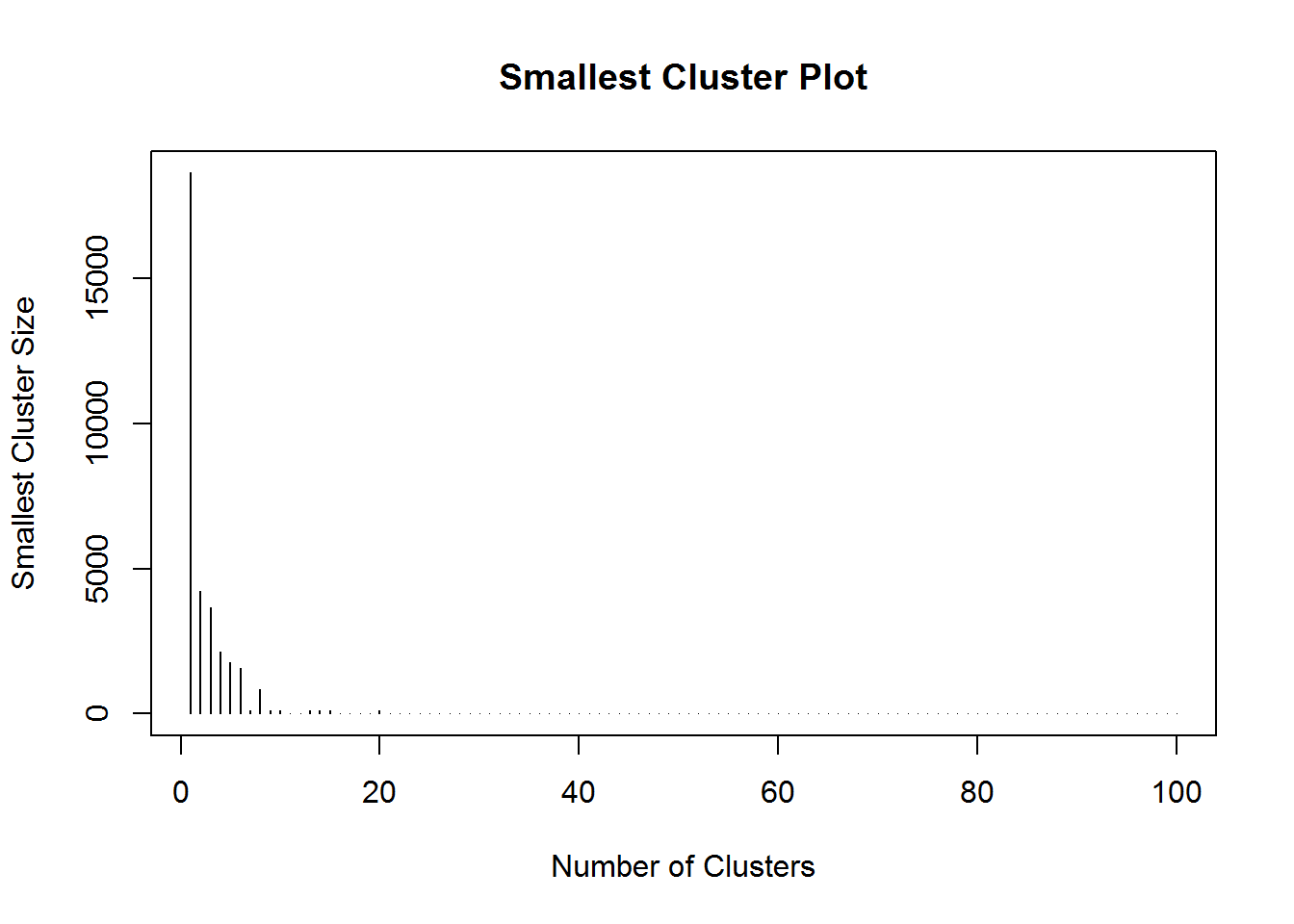When the number of the clusters is more than 8, it looks like very small clusters are being more common. Based on this info, using the cluster number 8, we can recalculate the classification. Note that the number of the cluster should be updated based on your own analysis. SAclus <- kmeans(PCA$scores[, 1:14], 8, iter.max = 100, nstart = 20)
SAclusters <- SAclus$cluster Diagnostics “Since the above computation applied the analysis to principal components it is helpful to characterise the clusters in terms of the original variables used in the PCA. To do this, firstly the ( z )-scores of each variable for the cluster centroids are computed. If the initial variable is ( x ), then the ( z )-score is given by $z = \frac{x - \bar{x}}{s}$ where ( {x} ) is the arithmetic mean of the ( x ) values in the data set, and ( s ) is the sample standard deviation defined by $s = + \sqrt{\frac{\sum{(x - \bar{x})^2}}{n - 1}}$ this standardisation allows values of each variable to be compared on a consistent scale - the ( z )-score in each case has a mean of zero and a standard deviation of one. Here, the ( x ) values are actually cluster-wise mean values, whereas ( {x} ) and ( s ) are computed for values for all Small Areas. This is useful for identifying which clusters have relatively high or low values of particular variables. The following code creates a set of ( z ) scores for each cluster" (Brunsdon et al., 2011). # We need this for the 'ddply' function library(plyr) # Compute a data frame (one row per cluster) containing the means of each variable in that cluster mean_by_cluster <- ddply(SAVars, .(SAclusters), numcolwise(mean))[, -1] # Compute the column-wise means for *all* observations mean_by_col <- apply(SAVars[, -1], 2, mean) # Compute the column-wise *sample* sd's for *all* observations sd_by_col <- apply(SAVars[, -1], 2, sd) # Create the z-scores via the 'scale' function z_scores <- scale(mean_by_cluster, center = mean_by_col, scale = sd_by_col) These values can be visualised to explore more and heatmap function can be one of the options. library(RColorBrewer) heatmap(t(z_scores), scale = 'none', col=brewer.pal(6,'BrBG'), breaks=c(-1e10,-2,-1,0,1,2,+1e10), xlab='Cluster Number', add.expr=abline(h=(0:40)+0.5,v=(0:6)+0.5,col='white'))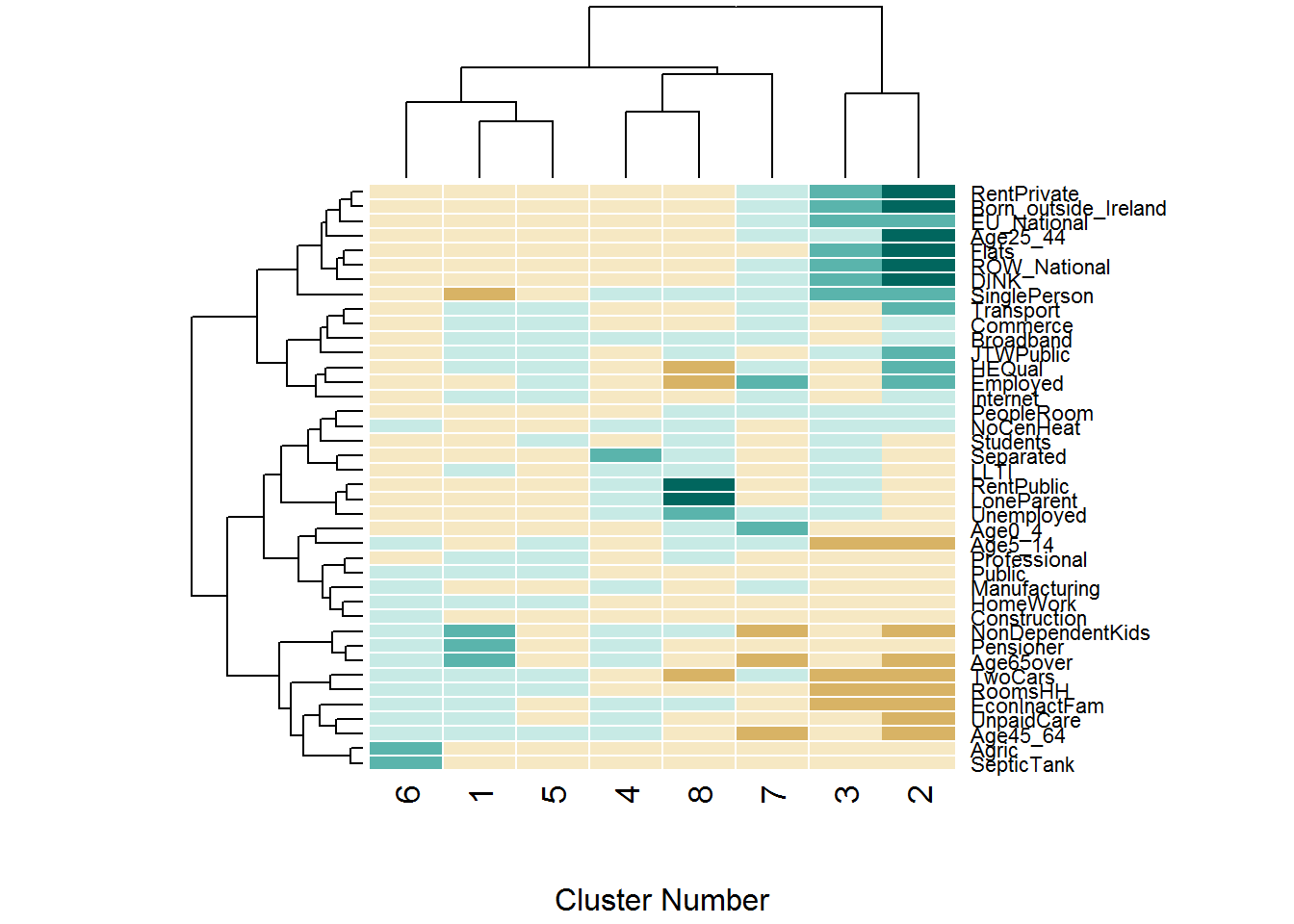We can also check the distribution of the size of each cluster group. barplot(sort(table(SAclusters)), las = 3)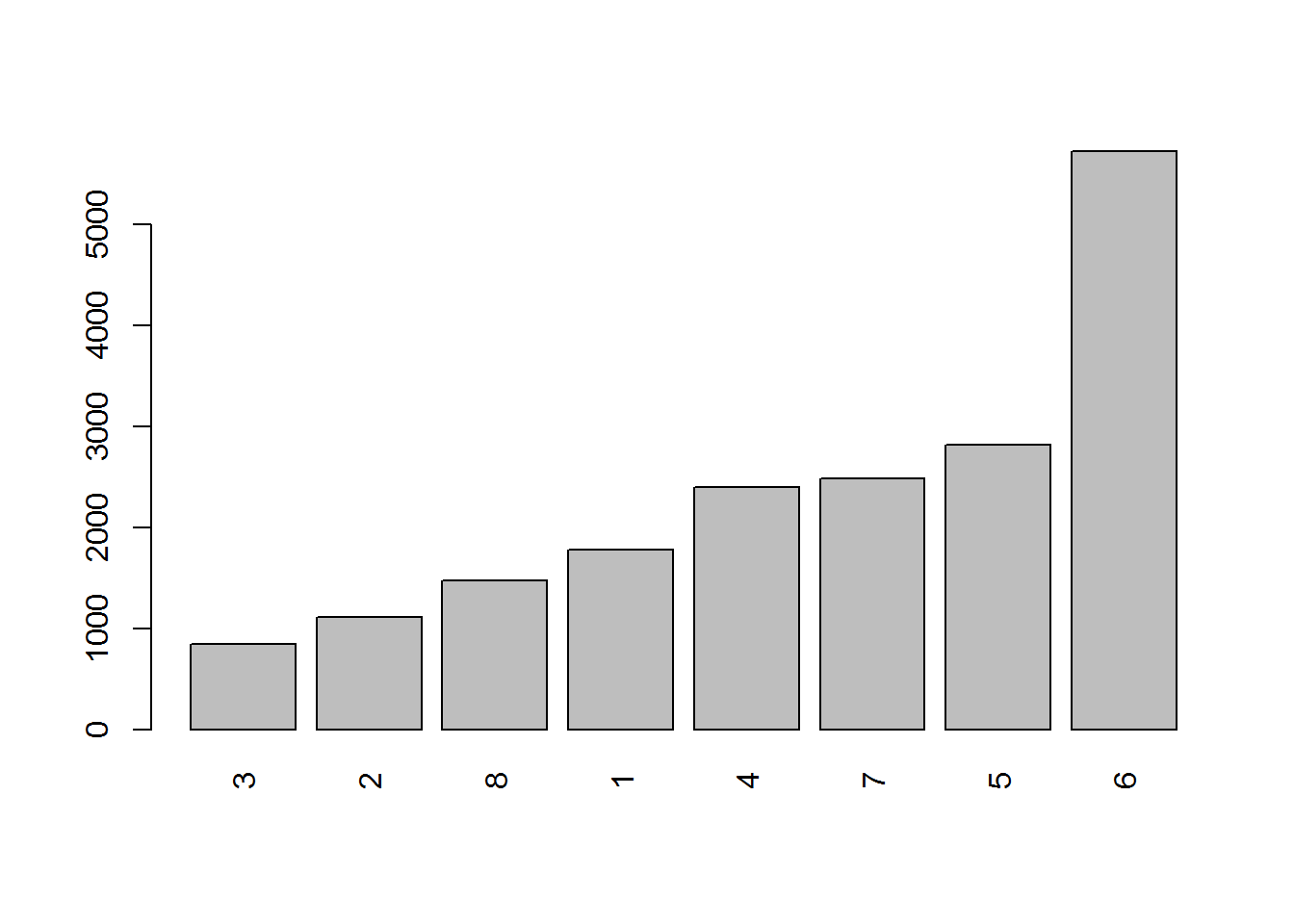Using PAM Instead of ( k )-Means As Brunsdon et al. ( 2011 ) mentioned the other alternative clustering technique is Kaufman and Rousseeuw’s Partitioning Around Medoids (PAM) algorithm ( 2 ). Note that the numbers can be different related to your own analysis results. After this stage, we will be repeating the previous steps; running z-score analysis, presenting the values by a heatmap and producing a barplot. library(cluster) PAMclus <- pam(x = PCA$scores[, 1:14], k = 8, pamonce = 2)
PAMclusters <- PAMclus\$clustering
mean_by_cluster2 <- ddply(SAVars, .(PAMclusters), numcolwise(mean))[, -1]
z_scores2 <- scale(mean_by_cluster2, center = mean_by_col, scale = sd_by_col)
heatmap(t(z_scores2),
scale = 'none',
col=brewer.pal(7,'BrBG'),
breaks=c(-1e10,-2,-1,-0.5,0.5,1,2,+1e10),
xlab='Cluster Number',
add.expr=abline(h=(0:40)+0.5,v=(0:6)+0.5,col='white'))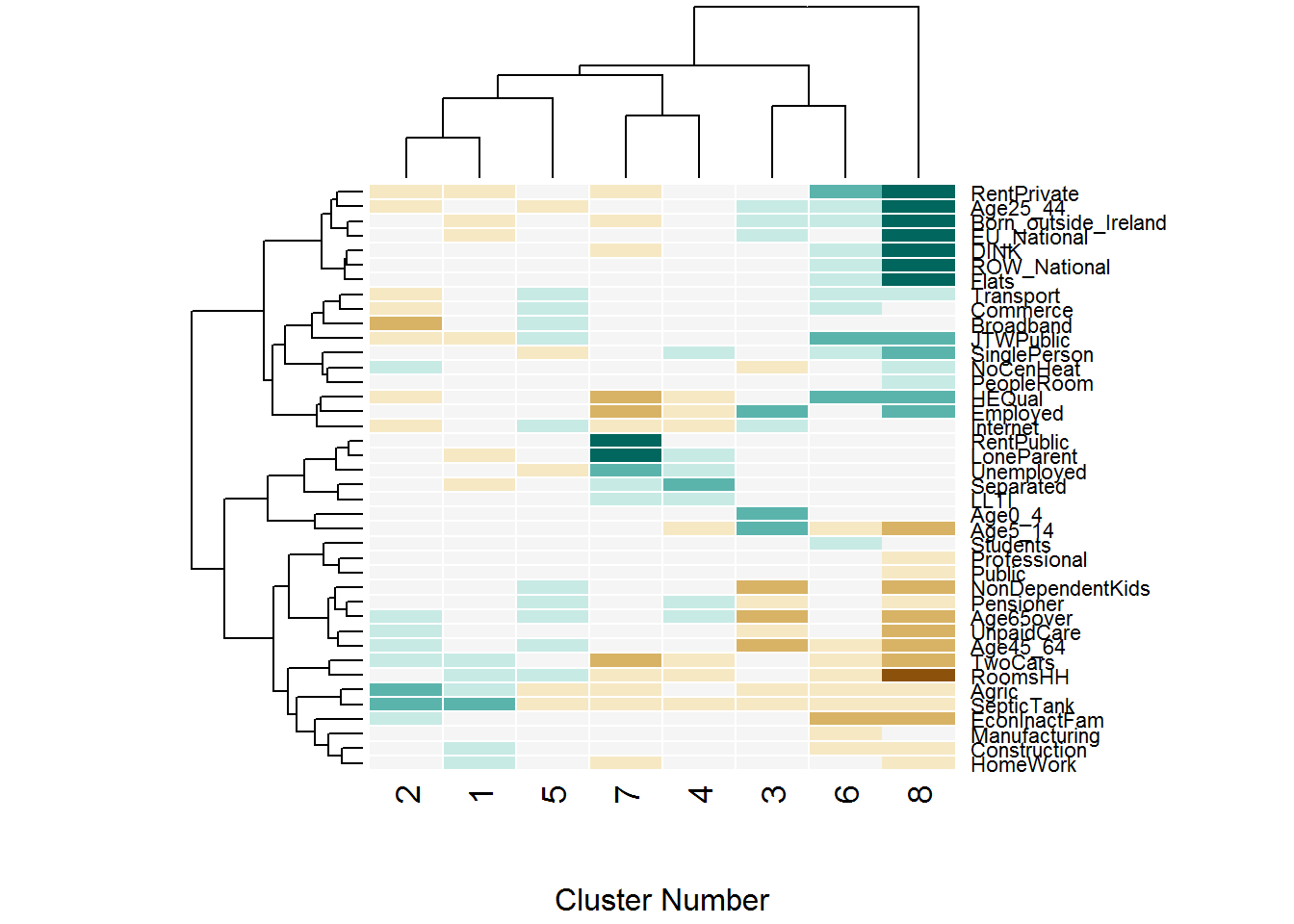barplot(sort(table(PAMclusters)), las = 3)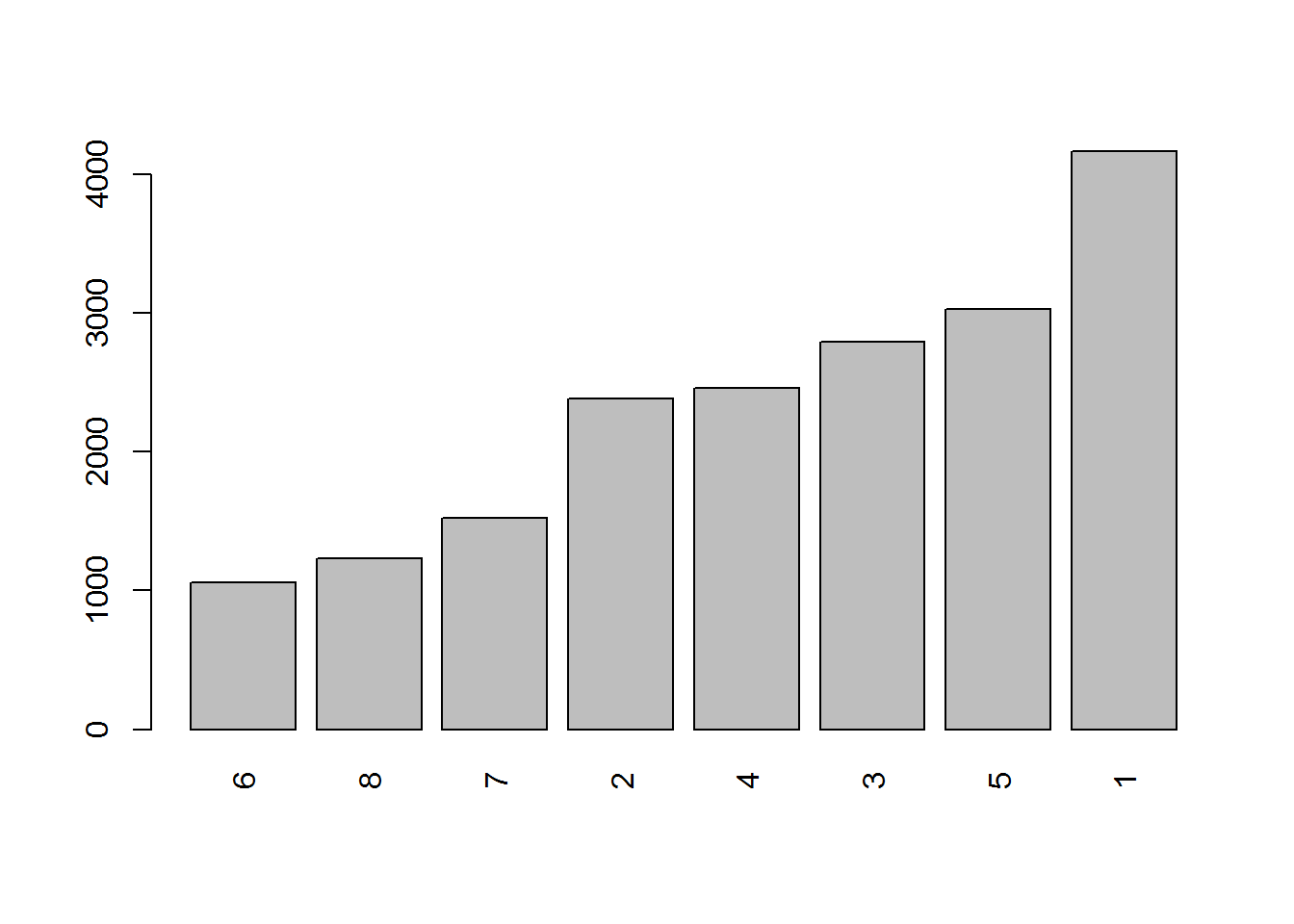Mapping Diagnostics

There are many possible ways to map your clusters. Some of the alternatives will be covered in this section.

#tmap option
library(tmap)
tm_shape(m)+
tm_fill("PAMclusters", title="clusters", legend.show=FALSE)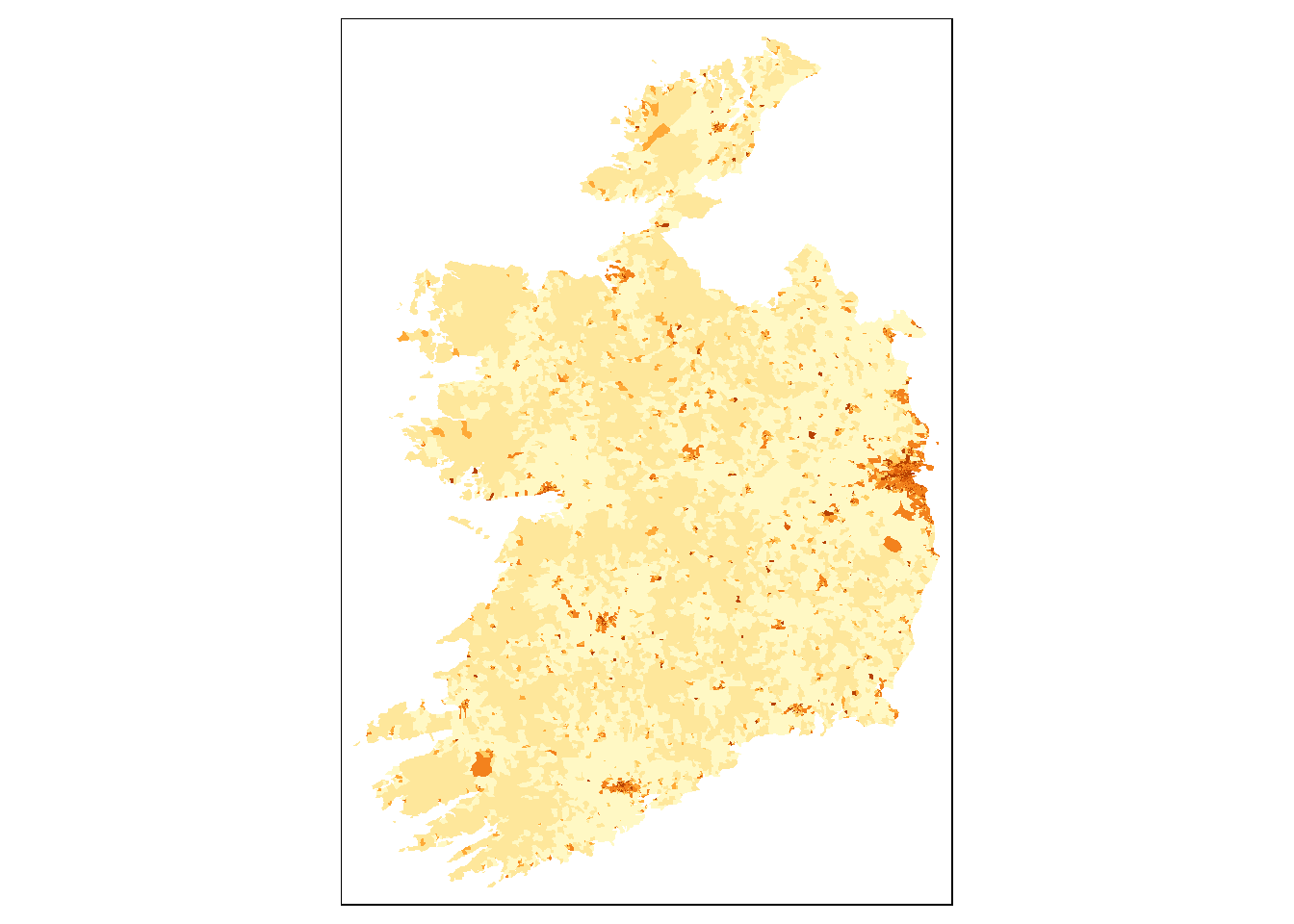#if you want to add facets as well
tm_shape(m)+
tm_fill("PAMclusters", title="clusters", legend.show=FALSE)+
tm_facets("PAMclusters")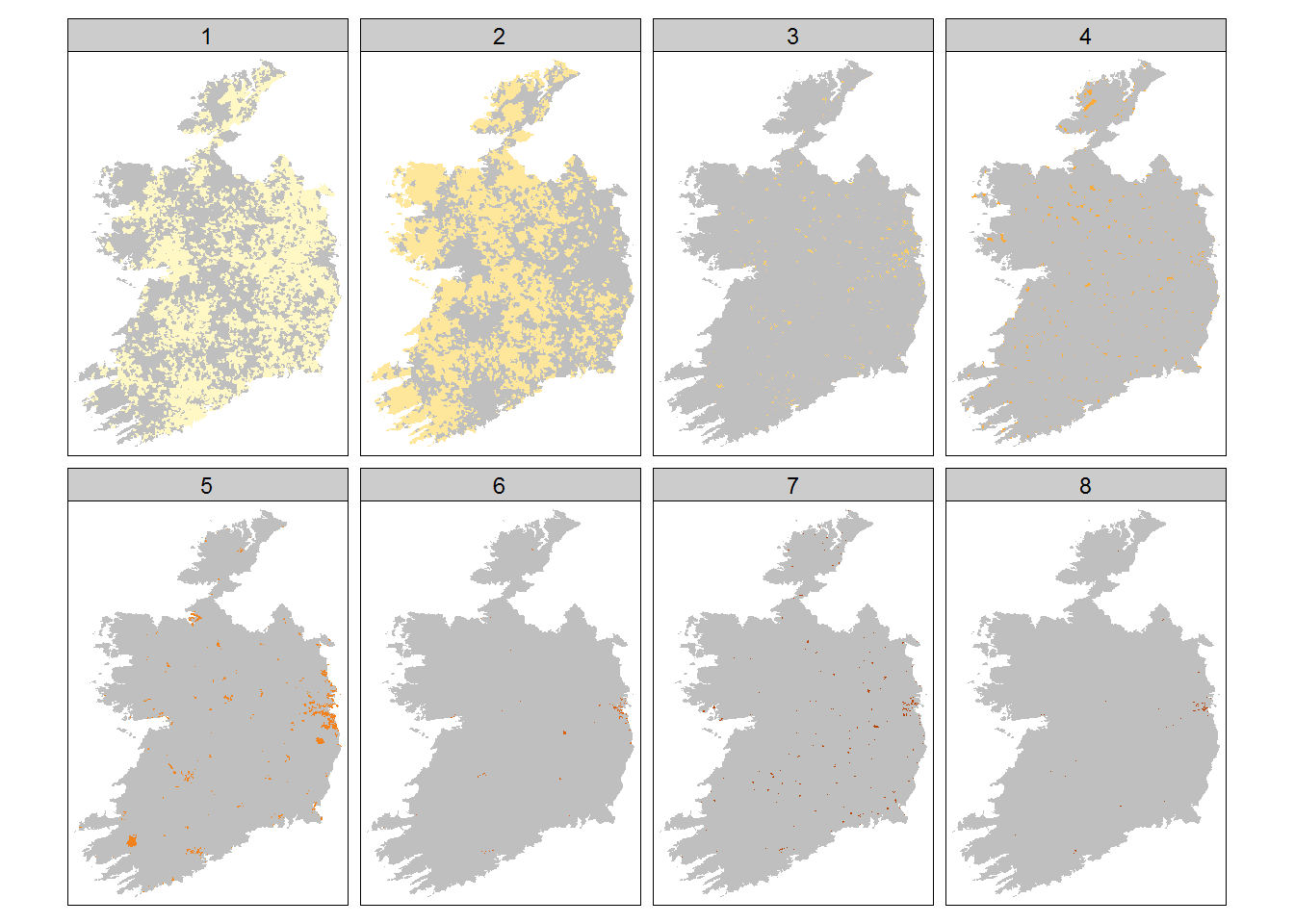Another alternative display style is Leaflet-Shiny integration. This is a useful tool when you want some interactivity for your map and data. Here are some images from the output.

You can see the live application through this link
Leaflet-Shiny example